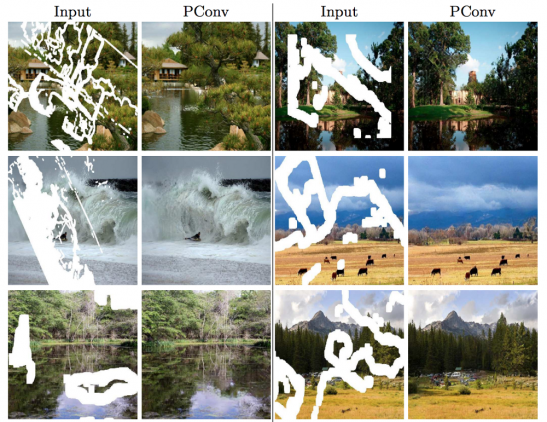• # Image Inpainting for Irregular Holes Using Partial Convolutions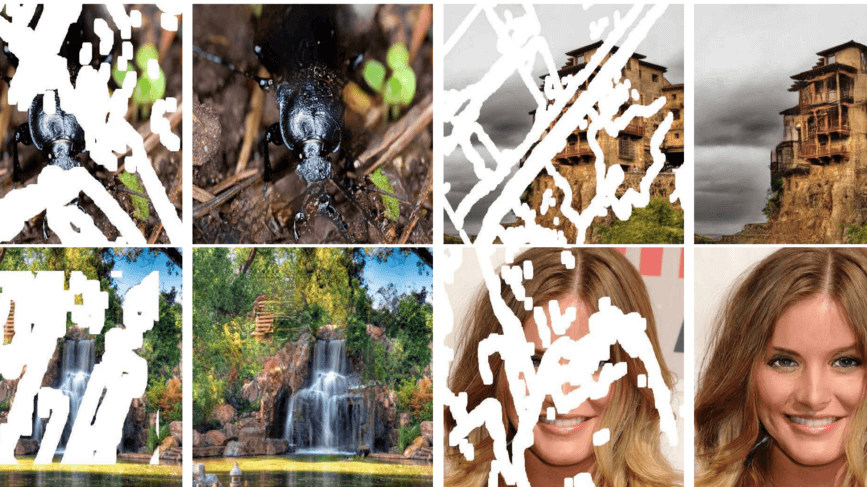Deep learning is growing very fast and it is one of the fast-growing areas of artificial intelligence. It has been used in many fields extensively including real-time object detection, image recognition, and video classification. Deep learning usually implemented as Convolutional Neural Network, Deep Belief Network, Recurrent Neural Network etc. One of the problems with images in image inpainting. Image inpainting is the task of filling the holes in an image. The goal of this work is to propose a model for image inpainting that operates robustly on irregular hole patterns and produces semantically meaningful predictions that incorporate smoothly with the rest of the image without the need for any additional post-processing or blending operation. It can be used with many applications e.g. it can be used in image editing to remove unwanted image content while filling the image with reasonable content.

There are many different approaches used in for image inpainting but none of them uses the deep learning approach and these approaches have some limitations. One of the methods is called patch match which iteratively searches for the best fitting patches to fill in the holes. While this approach generally produces smooth results, it is limited by the available image statistics and has no concept of visual semantics. Another limitation of many recent approaches is the focus on rectangular shaped holes, often assumed to be a center in the image. Another limitation of many recent methods is the focus on rectangular shaped holes, often assumed to be the center in the image. These limitations may lead to overfitting to the rectangular holes, and ultimately limit the utility of these models in the application.

## How Does It Work?

To overcome the limitations of the previous approach, Partial convolution has been used by Nvidia Research to solve the image inpainting problem. Partial Convolution Layer comprising a masked and re-normalized convolution operation followed by a mask-update step. The main extension is the automatic mask update step, which removes any masking where the partial convolution was able to operate on an unmasked value. The following contribution has been made:

• The use of partial convolutions with an automatic mask update step for achieving state-of-the-art on image inpainting.
• Substituting convolutional layers with partial convolutions and mask updates can achieve state-of-the-art inpainting results.
• Demonstrate the efficacy of training image-inpainting models on irregularly shaped holes.

## Partial Convolutional Layer

The model uses stacked partial convolution operations and mask updating steps to perform image inpainting. Partial convolution operation and mask update function jointly as the Partial Convolutional Layer.

Let W be the convolution filter weights for the convolution filter and b is the corresponding bias. is the feature values (pixels values) for the current convolution (sliding) window and M is the corresponding binary mask. The partial convolution at every location is expressed as: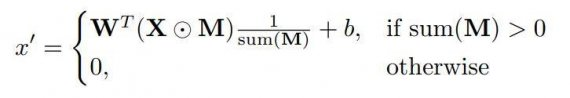Where ⊙ denotes element-wise multiplication. As can be seen, output values depend only on the unmasked inputs. The scaling factor 1/sum(M) applies appropriate scaling to adjust for the varying amount of valid (unmasked) inputs. After each partial convolution operation, the mask has been updated. The unmasking rule is simple: if the convolution was able to condition its output on at least one valid input value, then remove the mask for that location. This is expressed as:

and can easily be implemented in any deep learning framework as part of the forward pass.

## Network Architecture

Partial convolution layer is implemented by extending existing standard PyTorch. The straightforward implementation is to define binary masks of size C×H×W, the same size with their associated images/features, and then to implement mask updating is implemented using a fixed convolution layer, with the same kernel size as the partial convolution operation, but with weights identically set to 1 and bias set to 0. The entire network inference on a 512×512 image takes 0.23s on a single NVIDIA V100 GPU, regardless of the hole size.

The architecture used is UNet-like architecture, replacing all convolutional layers with partial convolutional layers and using nearest neighbor up-sampling in the decoding stage.Figure 1: This architecture used in image inpainting where all the convolutional layers replaced with partial convolutional layers

ReLU is used in the encoding stage and LeakyReLU with alpha = 0.2 is used between all decoding layers. The encoder comprises eight partial convolutional layers with stride=2. The kernel sizes are 7, 5, 5, 3, 3, 3, 3 and 3. The channel sizes are 64, 128, 256, 512, 512, 512, 512, and 512. The last partial convolution layer’s input will contain the concatenation of the original input image with hole and original mask.

## Loss Function

The loss functions target both per-pixel reconstruction accuracy as well as composition, i.e. how smoothly the predicted hole values transition into their surrounding context. Given an input image with hole Iin and mask M, the network prediction Iout and ground truth image Igt, then the pixel loss is defined as: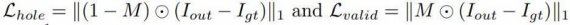The perceptual (perceptual loss functions measures high-level perceptual and semantic differences between images. They make use of a loss network which is pretrained for image classification, meaning that these perceptual loss functions are themselves deep convolutional neural networks) loss is defined as: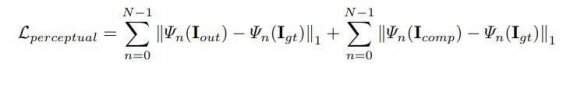The perceptual loss computes the L1 distances between both Iout and Icompand the ground truth. To perform autocorrelation, style loss term is introduced on each feature map.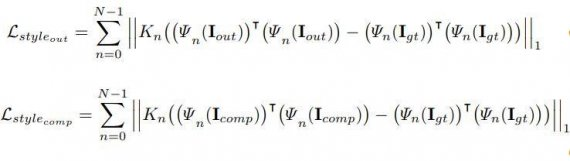The total loss is the combination of all the above loss: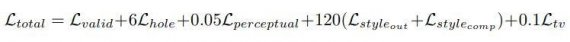## Results

Partial convolution outperforms other methods. To prove that partial convolution performs better than other methods, l1 error, peak signal-to-noise ratio (PSNR), Structural SIMilarity (SSIM) index and Inception score(IScore) evaluation metrics are used. Below table shows the comparison results. It can be seen that PConv method outperforms all the other methods on these measurements on irregular masks.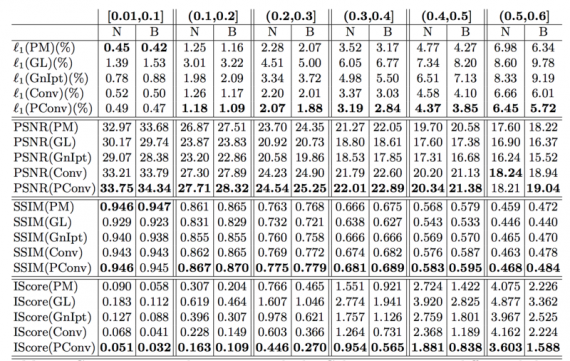The use of a partial convolution layer with an automatic mask updating mechanism and achieve state-of-the-art image inpainting results. The model can robustly handle holes of any shape, size location, or distance from the image borders. Further, the performance does not deteriorate catastrophically as holes increase in size, as seen in Figure 2.